# Graph Theory

## Introduction

Graph data structures are among the most important and exciting topics to learn in computer science because of their usage in several real-world applications like in Google Maps, Circuit Designing, etc.

Formally, A graph is a non-linear data structure containing nodes and links between them called edges.In this article, we will see various graph terminologies, important theorems, and some special simple graphs.

Before we proceed, have you considered how we might represent these graphs?

There are two common ways we use to represent graphs, and the two ways are as follows :

## Terminologies in Graph

Basic graph terminology, such as vertices, edges, directed and undirected edges, and so on, have already been covered in this blog. Some additional terms to describe the properties of graphs are as follows:

1. Degree of a node: The degree of a node is the number of edges incident to that node. The degree of vertex u is denoted by deg(u).

Note: A vertex with a degree 0 is called isolated. The one with degree 1 is called a pendant.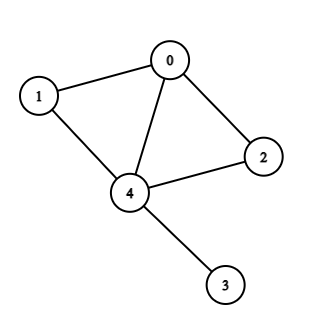The degree of the nodes in the above-undirected graph is given in the table below:

• Indegree: denoted by deg-(v) is the number of edges incident to that node.
• Outdegree: denoted by deg+(v) is the number of edges outgoing from that node. The degree of a node is the sum of outdegree and indegree.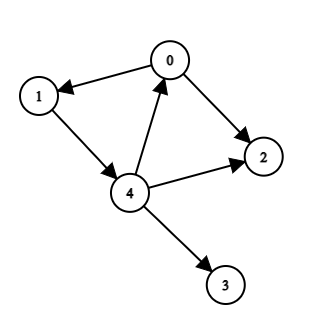The indegree and outdegree of the above graph are given in the table below:

2. Path: A path is defined as a sequence of nodes in the order we're visiting them. The order in which we go from node 'u' to reach v is known as path.

Note: No node should be repeated in the path ever, except when the source and destination are the same.

Here, 2 -> 4 -> 0 -> 1 is a path from vertex 2 to 1.

3. Walk: A walk is a sequence of vertices and edges of a graph. In a walk, vertices and edges can be repeated.

Here, 1 -> 0 -> 2 -> 3 -> 2 -> 4 is a walk.

• Open walk: A walk is an open walk when the initial and final vertices are different.

For example: In the above graph, 1 -> 0 -> 2 -> 4 -> 3 is an open walk.

• Closed walk: A walk is an open walk when the initial and final vertices are the same.

For example: In the above graph, 1 -> 0 -> 2 -> 4 ->1 is a closed walk.

4. Trail: An open walk in which no edge is repeated is called a trail.

Note: Vertex can be repeated in a trail.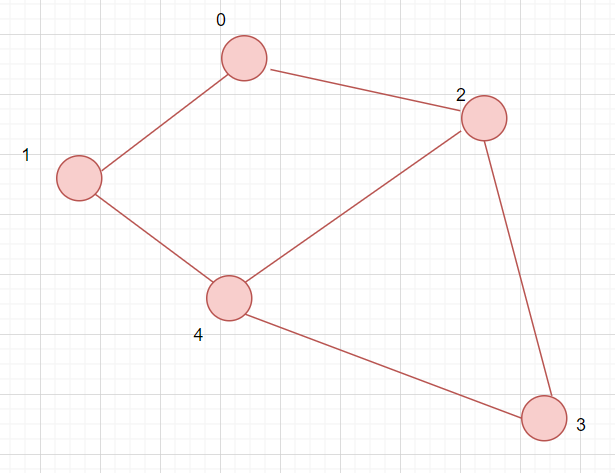In the above example, 1 -> 0 -> 2 -> 3 -> 4 -> 2 is a trail. Here, vertex 2 is repeated but not any edge.

5. Circuit: A graph traversal that is closed and in which there is no edge repetition, but vertex repetition can occur called a circuit.In the above example, 1 -> 0 -> 2 -> 3 -> 4 ->1 is a circuit. Here, vertex 1 is repeated but not any edge.

## Important theorems in Graph theory

Graphs are classified as directed or undirected based on the type of edges, and there are several subcategories within each (Refer to the diagram below). There are various mathematical theorems associated with graphs in graph theory. We’ll see some of them in detail.

Let's look at some essential theorems in graphs.

1. Handshaking Theorem :

"In an undirected graph, the sum of degrees of all the vertices equals twice the number of edges".

Mathematically, Let G = (V,E) be an undirected graph with e edges. Then, 2e = ∑u∈V deg(u).In the case of an directed graph, ∑u∈V deg-(u) = ∑u∈V deg+(u) = |E|.

Proof: We know that the degree of a vertex is the number of edges incident on it. So, when we find out the sum of degrees, we're counting each edge twice because, in an undirected graph, one edge is incident on two vertices. Therefore, each edge gets counted twice. Thus, the sum of degrees is twice the number of edges.

In the case of a directed graph, the edges don’t get counted twice because they are the only incident on one of the two vertices they’re connecting. So, each edge is counted once. Also, we know that the number of incoming edges is equal to the number of outgoing edges because each edge is incident on one vertex and outgoing from one edge.The handshaking theorem is applicable even in the cases of multiple edges and loops.

Corollary:

An undirected graph has an even number of vertices of odd degrees.

Proof: Let v1 be the number of vertices with even degrees and v2 be the number of vertices with odd degrees.

We know, from the handshaking theorem,
2e = ∑u∈V deg(u) implies,
2e = ∑u∈V1 deg(u) + ∑u∈V2 deg(u)

The sum of degrees of vertices with even degrees is even. Since the LHS is even for sure, and the first part of the RHS is even, so the sum of degrees of vertices with odd degrees must be even. Thus, the number of vertices with odd degrees is even.

2. Bipartite theorem:

“A simple graph is bipartite if and only if it is possible to assign one of two different colors to each vertex of the graph and no two adjacent vertices are assigned the same color".

Proof: A bipartite graph is a graph whose vertices can be divided into two disjoint sets such that every edge in G has its initial vertex in the first set and the terminal vertex in the second set.

So, if we start coloring the vertices of a bipartite graph since the vertices of an edge can be divided into two disjoint sets, in this case, they can be colored by two different colors. We're doing this for every edge, so no two vertices will have the same color this way.

1. “A complete graph has n*(n-1)/2 edges”.

Proof: A complete graph is a graph in which every vertex is connected to every other vertex. If there are a total of n vertices, and we take one, there are other n-1 vertices that it will be connected to.

So, you have n-1 outgoing edges from this first vertex. The second vertex that we'll choose has already connected with the first vertex, so it'll have outgoing edges to other n-2 vertices.

In general, the kth vertex has already been connected to previous k-1 vertices. Therefore, it'll have n-k outgoing edges with the remaining n-k vertices. So, the total number of edges is:

(n-1) + (n-2) + .... + 2 + 1  =  n * (n-1) / 2

Source: Wikimedia

The diagram above contains a complete graph of 5 vertices, and it has 5*(5-1)/2 = 10 edges.

## Simple Special Graphs

1. Cycles: The graphs with vertices n>=3 and edges {1,2},{2,3}...{n-1,n} and {n,1} are called cycles. The total number of edges in a cyclic graph of n vertices is n.

2. Wheels: A wheel is very similar to cycles, other than the fact that they consist of one additional vertex connected to every other vertex. The total number of edges in a wheel of n vertices is 2*(n-1).

3. Hypercube: A graph where vertices are represented as an n-bit string ( like their binary representation). The vertices, which differ by at most 1-bit, are connected by edges.  The total edges in a hypercube of 2^n vertices are n*(2^(n-1)).

Source: Wikipedia

1. Subgraph: A graph G1 = (V1, E1) is said to be the subgraph of another graph G(V, E) if every vertex V1(G1)  is in the graph G(V, E) and each of the edges E1(G1) is a subset of E(G) such that each edge of G1 has the same two end vertices as G.

For example, here, G1 is a subgraph of G.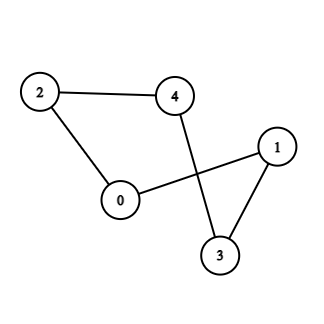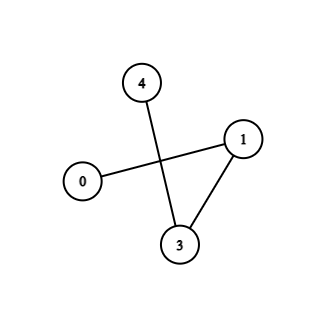G G1

1. What is a graph?
Answer: A graph is a mathematical representation of a network, and it describes the relationship between lines and points.

2. What does graph theory teach us about?
Answer: Graph theory is the study of relationships. Graph theory is a helpful tool for quantifying and simplifying the various moving aspects of dynamic systems, given a set of nodes and connections that can abstract anything from city plans to computer data.

3. What are the properties of graph theory?
Answer: A graph is a data structure made up of nodes and edges that are non-linear. The edges are lines or arcs that connect any two nodes in the graph, and the nodes are also known as vertices.

4. What is the fundamental difference between a tree and a graph?
Answer: tree is a special kind of graph that contains no cycles. So, every tree is a graph, but all graphs are not trees.

5. What are the real-life applications of graphs?
Answer: Graphs represent social groups like in facebook's graph API, in making knowledge graphs, in path optimization algorithms for finding the shortest path between points, in GPS navigation systems, and chemistry.

## Key Takeaways

We discussed some of the fundamental theorems here in this article. You can look for more similar articles on graph theory as an introduction to graphs and graph representation here. Once you learn all the basics, you’re good to go and solve graph-related problems.

Problems related to graphs are asked during various coding contests as well as placements tests.

To practice and learn more about such topics, Codestudio is a one-stop destination. This platform will help you acquire effective coding techniques and an overview of interview experiences of various product-based companies.

Happy Coding!